# NCERT solutions for Physics Part 1 and 2 Class 12 chapter 14 - Semiconductor Electronics: Materials, Devices and Simple Circuits [Latest edition]

#### Chapters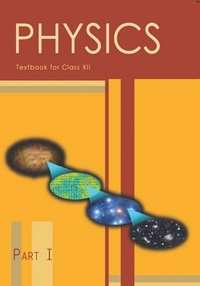## Chapter 14: Semiconductor Electronics: Materials, Devices and Simple Circuits

Exercise
Exercise [Pages 497 - 499]

### NCERT solutions for Physics Part 1 and 2 Class 12 Chapter 14 Semiconductor Electronics: Materials, Devices and Simple Circuits Exercise [Pages 497 - 499]

Exercise | Q 14.1 | Page 497

In an n-type silicon, which of the following statement is true:

• Electrons are majority carriers and trivalent atoms are the dopants.

• Electrons are minority carriers and pentavalent atoms are the dopants.

• Holes are minority carriers and pentavalent atoms are the dopants.

• Holes are majority carriers and trivalent atoms are the dopants.

Exercise | Q 14.2 | Page 497

In a p-type semiconductos, which of the following statement is true:

• Electrons are majority carriers and trivalent atoms are the dopants.

• Electrons are minority carriers and pentavalent atoms are the dopants.

• Holes are minority carriers and pentavalent atoms are the dopants.

• Holes are majority carriers and trivalent atoms are the dopants.

Exercise | Q 14.3 | Page 497

Carbon, silicon and germanium have four valence electrons each. These are characterised by valence and conduction bands separated by energy band gap respectively equal to (Eg)C, (Eg)Si and (Eg)Ge. Which of the following statements is true?

• (Eg)Si < (Eg)Ge < (Eg)C

• (Eg)C < (Eg)Ge > (Eg)Si

• (Eg)C > (Eg)Si > (Eg)Ge

• (Eg)C = (Eg)Si = (Eg)Ge

Exercise | Q 14.4 | Page 497

In an unbiased p-n junction, holes diffuse from the p-region to n-region because ______.

• free electrons in the n-region attract them.

• they move across the junction by the potential difference.

• hole concentration in p-region is more as compared to n-region.

• All the above.

Exercise | Q 14.5 | Page 467

When a forward bias is applied to a p-n junction, it ______.

• raises the potential barrier.

• reduces the majority carrier current to zero.

• lowers the potential barrier.

• None of the above.

Exercise | Q 14.6 | Page 497

In half-wave rectification, what is the output frequency if the input frequency is 50 Hz. What is the output frequency of a full-wave rectifier for the same input frequency.

Exercise | Q 14.7 | Page 497

A p-n photodiode is fabricated from a semiconductor with band gap of 2.8 eV. Can it detect a wavelength of 6000 nm?

Exercise | Q 14.8 | Page 498

The number of silicon atoms per m3 is 5 × 1028. This is doped simultaneously with 5 × 1022 atoms per m3 of Arsenic and 5 × 1020 per m3 atoms of Indium. Calculate the number of electrons and holes. Given that ni= 1.5 × 1016 m−3. Is the material n-type or p-type?

Exercise | Q 14.9 | Page 498

In an intrinsic semiconductor the energy gap Egis 1.2 eV. Its hole mobility is much smaller than electron mobility and independent of temperature. What is the ratio between conductivity at 600K and that at 300K? Assume that the temperature dependence of intrinsic carrier concentration niis given by

"n"_"i" = "n"_0 exp(- "E"_"g"/(2"k"_"BT"))

where nis a constant.

Exercise | Q 14.10 | Page 497

In a p-n junction diode, the current I can be expressed as

I = "I"_0 exp ("eV"/(2"k"_"BT") - 1)

where I0 is called the reverse saturation current, V is the voltage across the diode and is positive for forward bias and negative for reverse bias, and I is the current through the diode, kBis the Boltzmann constant (8.6×10−5 eV/K) and T is the absolute temperature. If for a given diode I0 = 5 × 10−12 A and T = 300 K, then

(a) What will be the forward current at a forward voltage of 0.6 V?

(b) What will be the increase in the current if the voltage across the diode is increased to 0.7 V?

(c) What is the dynamic resistance?

(d) What will be the current if reverse bias voltage changes from 1 V to 2 V?

Exercise | Q 14.11 | Page 498

You are given the two circuits as shown in following figure. Show that circuit

1. acts as OR gate while the circuit
2. acts as AND gate.

(a)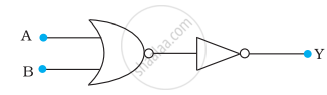(b)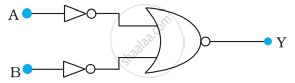Exercise | Q 14.12 | Page 498

Write the truth table for a NAND gate connected as given in the following figure.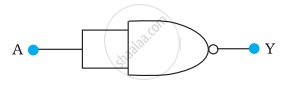Hence identify the exact logic operation carried out by this circuit

Exercise | Q 14.13 | Page 499

You are given two circuits as shown in following figure, which consist of NAND gates. Identify the logic operation carried out by the two circuits.

(a)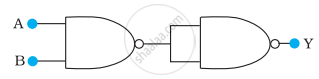(b)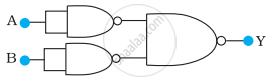Exercise | Q 14.14 | Page 499

Write the truth table for circuit given in figure below consisting of NOR gates and identify the logic operation (OR, AND, NOT) which this circuit is performing.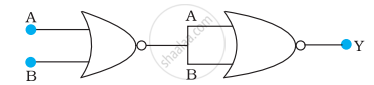(Hint: A = 0, B = 1 then A and B inputs of second NOR gate will be 0 and hence Y=1. Similarly work out the values of Y for other combinations of A and B. Compare with the truth table of OR, AND, NOT gates and find the correct one.)

Exercise | Q 14.15 | Page 499

Write the truth table for the circuits given in following figure consisting of NOR gates only. Identify the logic operations (OR, AND, NOT) performed by the two circuits.

(a)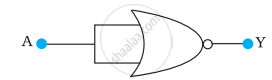(b)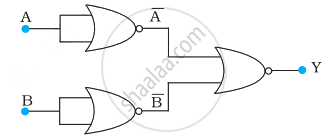## Chapter 14: Semiconductor Electronics: Materials, Devices and Simple Circuits

Exercise## NCERT solutions for Physics Part 1 and 2 Class 12 chapter 14 - Semiconductor Electronics: Materials, Devices and Simple Circuits

NCERT solutions for Physics Part 1 and 2 Class 12 chapter 14 (Semiconductor Electronics: Materials, Devices and Simple Circuits) include all questions with solution and detail explanation. This will clear students doubts about any question and improve application skills while preparing for board exams. The detailed, step-by-step solutions will help you understand the concepts better and clear your confusions, if any. Shaalaa.com has the CBSE Physics Part 1 and 2 Class 12 solutions in a manner that help students grasp basic concepts better and faster.

Further, we at Shaalaa.com provide such solutions so that students can prepare for written exams. NCERT textbook solutions can be a core help for self-study and acts as a perfect self-help guidance for students.

Concepts covered in Physics Part 1 and 2 Class 12 chapter 14 Semiconductor Electronics: Materials, Devices and Simple Circuits are Concept of Semiconductor Electronics: Materials, Devices and Simple Circuits, Integrated Circuits, Feedback Amplifier and Transistor Oscillator, Transistor as a Device, Basic Transistor Circuit Configurations and Transistor Characteristics, Application of Junction Diode as a Rectifier, p-n Junction, Intrinsic Semiconductor, Classification of Metals, Conductors and Semiconductors, Extrinsic Semiconductor, Transistor Action, Transistor: Structure and Action, Digital Electronics and Logic Gates, Transistor as an Amplifier (Ce-configuration), Transistor and Characteristics of a Transistor, Semiconductor Diode, Zener Diode as a Voltage Regulator, Special Purpose P-n Junction Diodes, Diode as a Rectifier, Energy Bands in Conductors, Semiconductors and Insulators, Triode.

Using NCERT Class 12 solutions Semiconductor Electronics: Materials, Devices and Simple Circuits exercise by students are an easy way to prepare for the exams, as they involve solutions arranged chapter-wise also page wise. The questions involved in NCERT Solutions are important questions that can be asked in the final exam. Maximum students of CBSE Class 12 prefer NCERT Textbook Solutions to score more in exam.

Get the free view of chapter 14 Semiconductor Electronics: Materials, Devices and Simple Circuits Class 12 extra questions for Physics Part 1 and 2 Class 12 and can use Shaalaa.com to keep it handy for your exam preparation